01

## 圆锥曲线与密切圆交点几何性质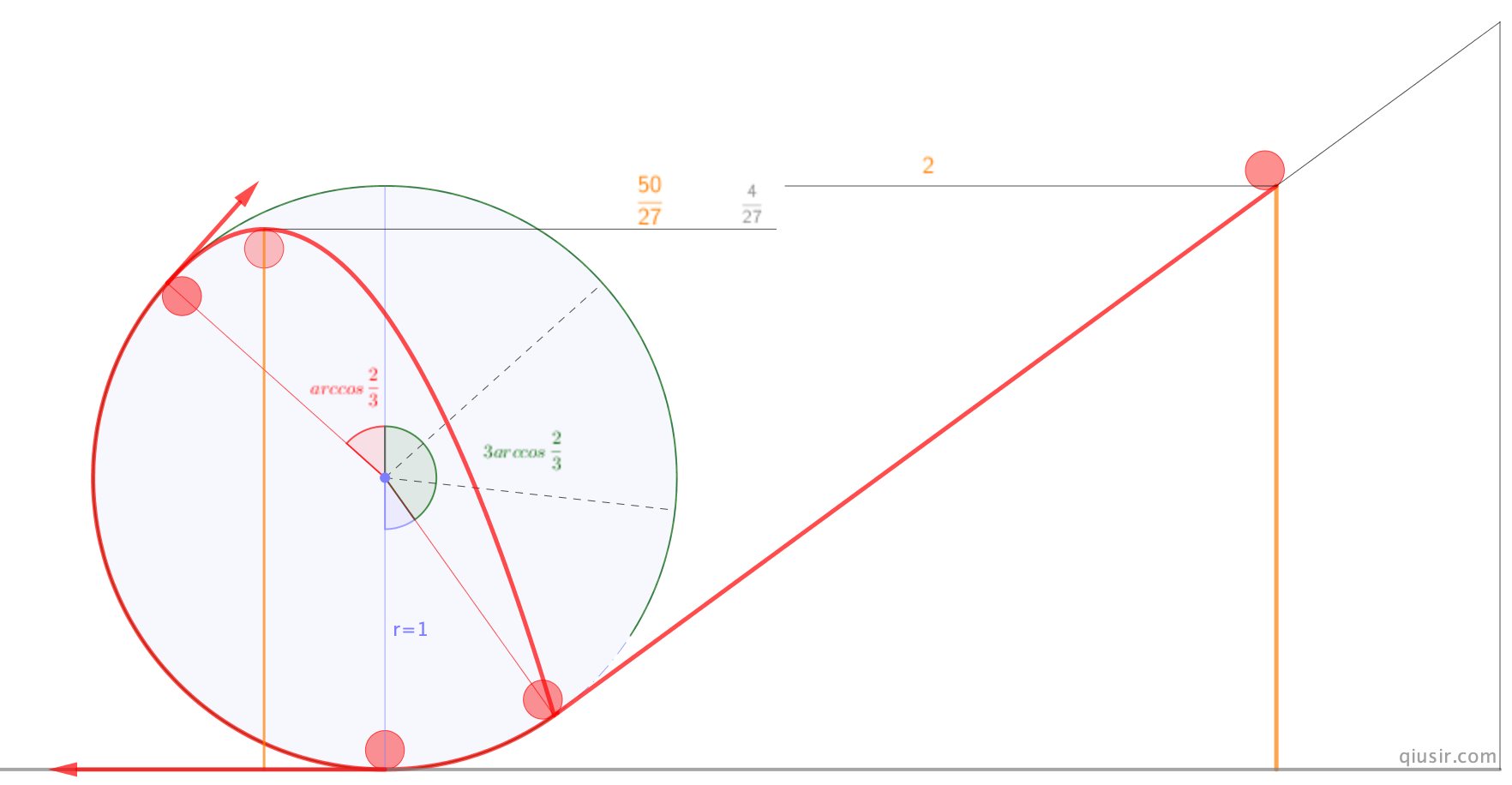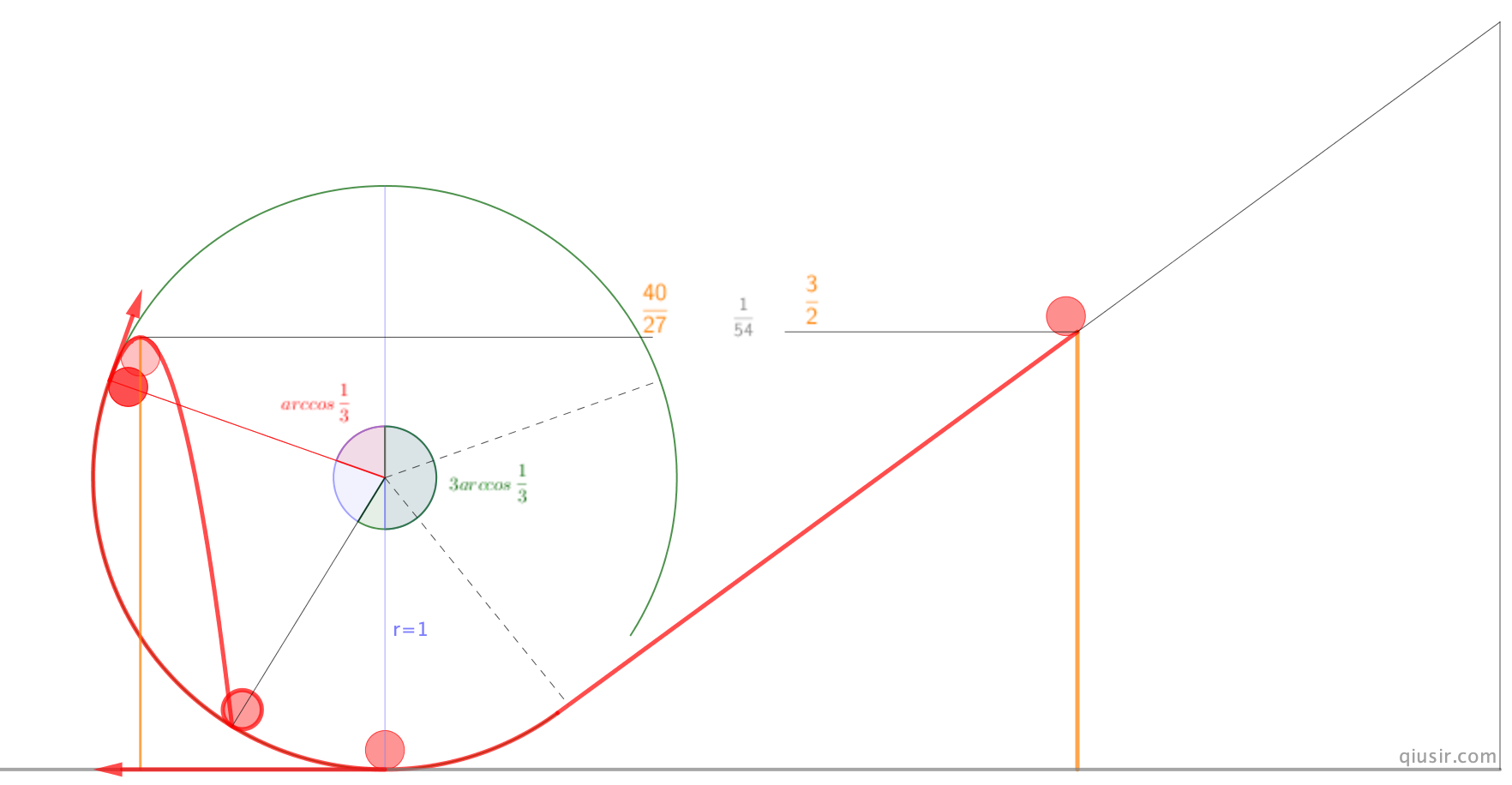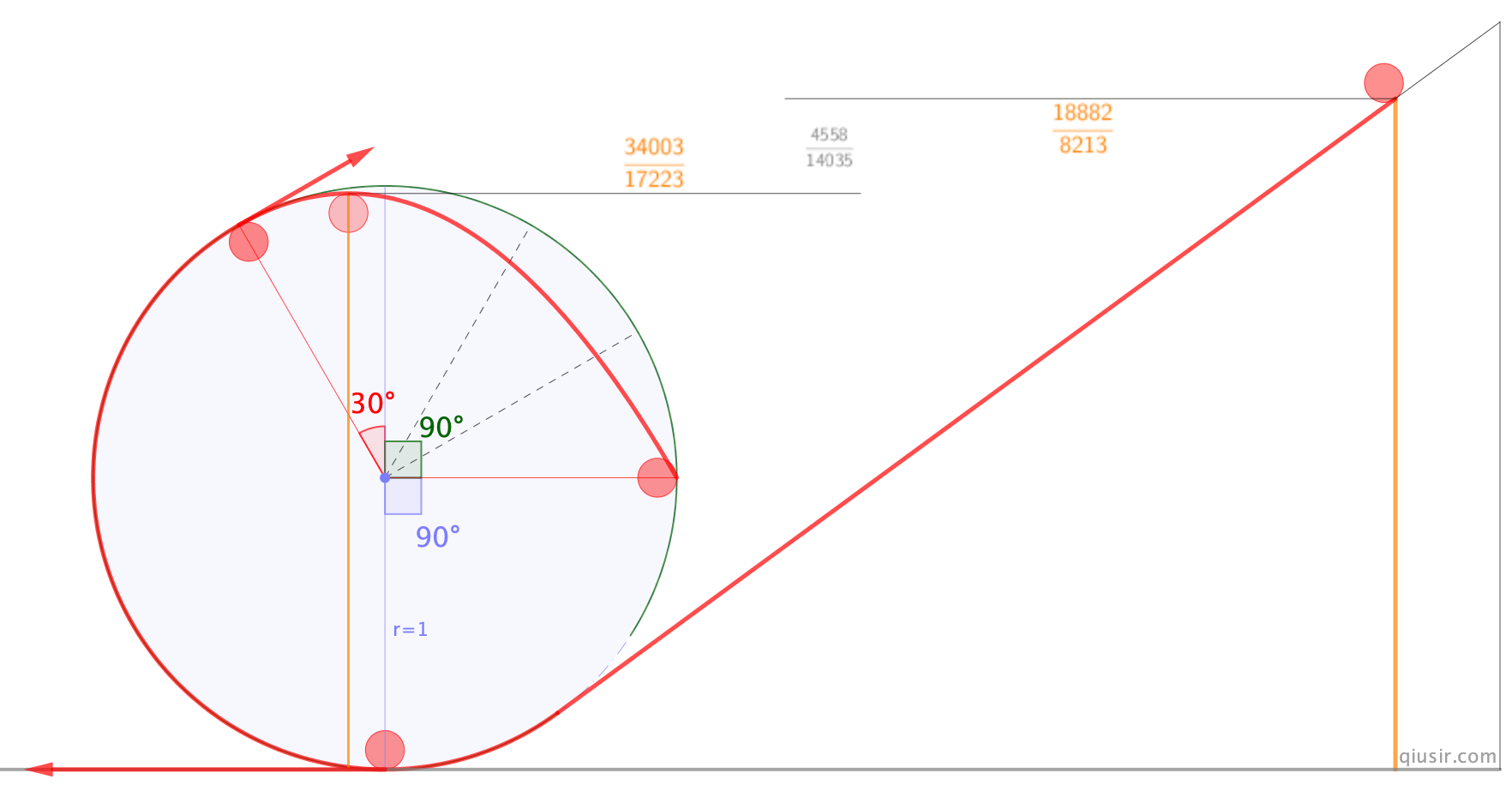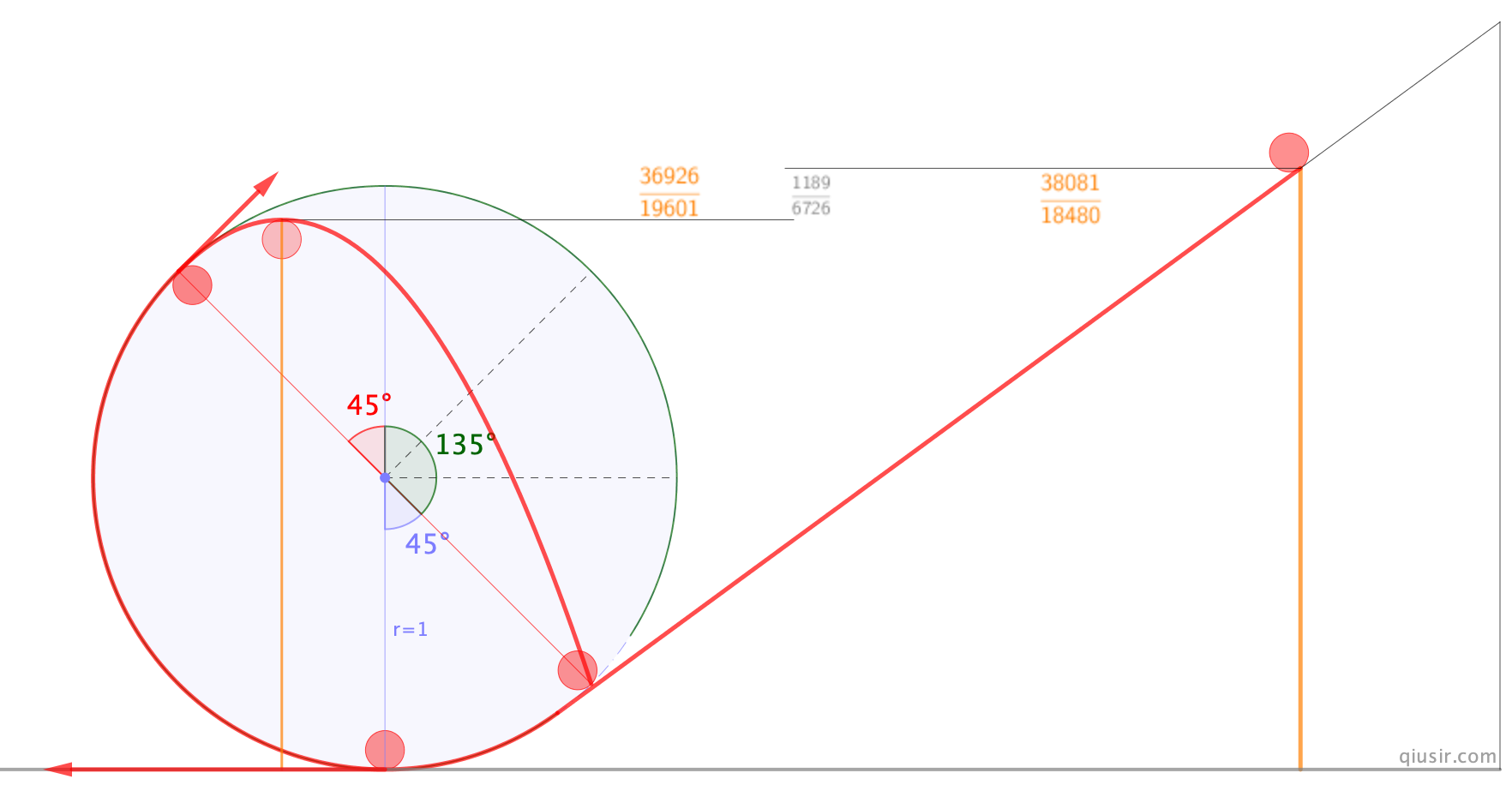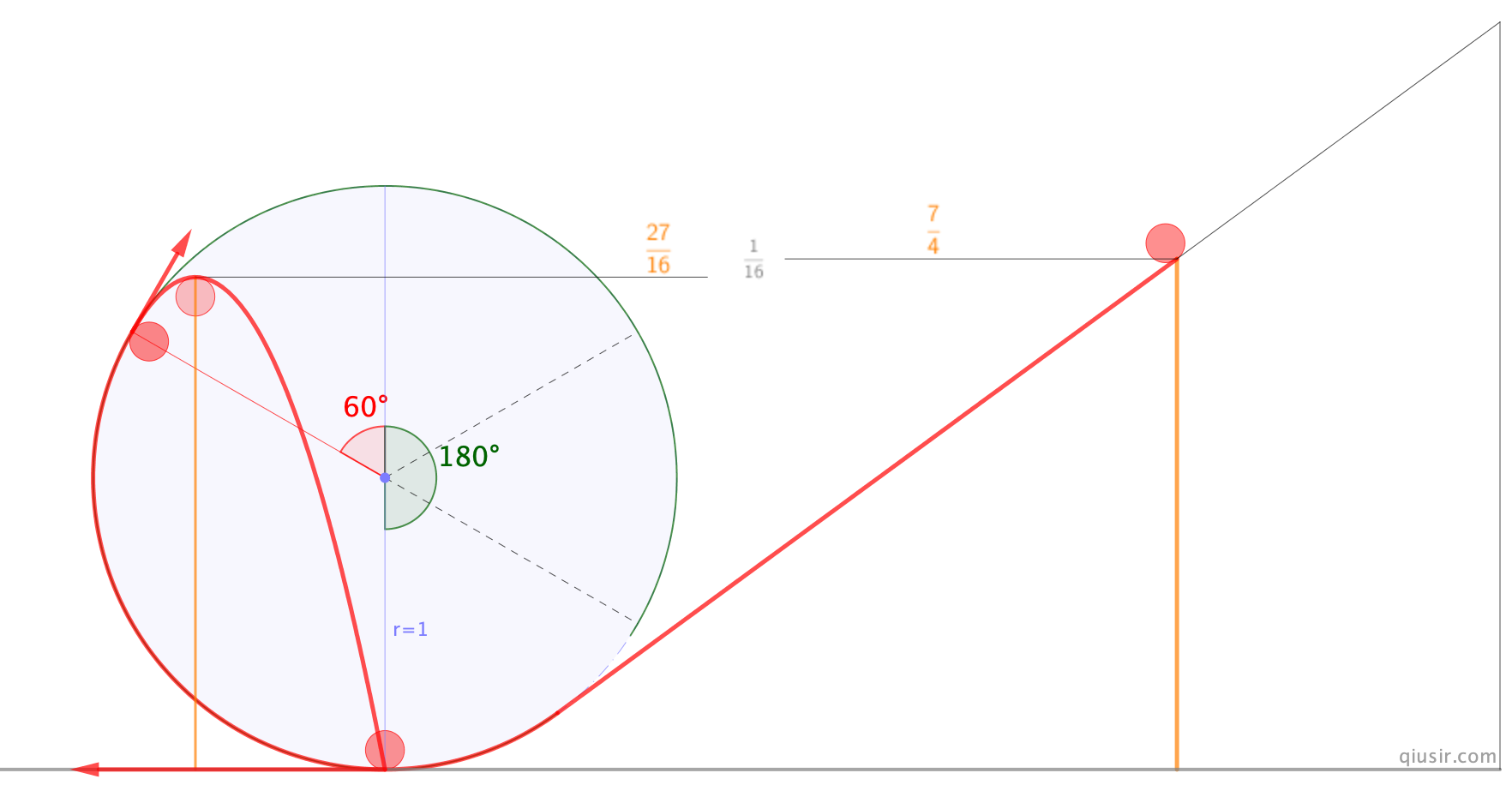update:

$v_x=vsin(\frac{\pi}{2}-2\alpha)$$g_x=gsin\alpha$
$v_y=vcos(\frac{\pi}{2}-2\alpha)$$g_y=gcos\alpha$
$t=2\frac{v_y}{g_y}=\frac{4vsin\alpha}{g}$
$x=v_xt+\frac{1}{2}g_xt^2$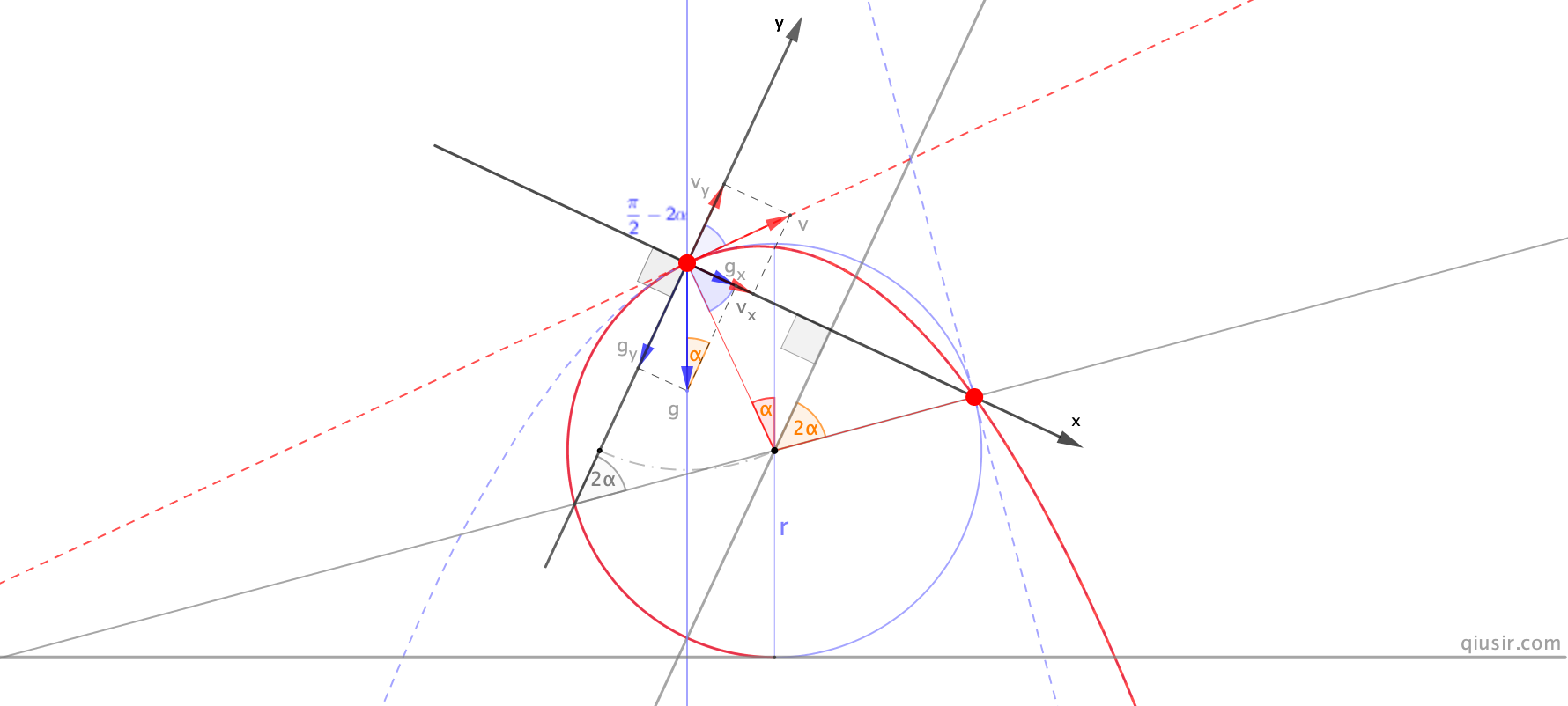1、构造过分离点A的竖直直线，也是重力方向，与圆相交B点；2、连接B与圆心的直线；3、过分离点A做垂线，与圆相交C即为落点。也是A关于直线的对称点。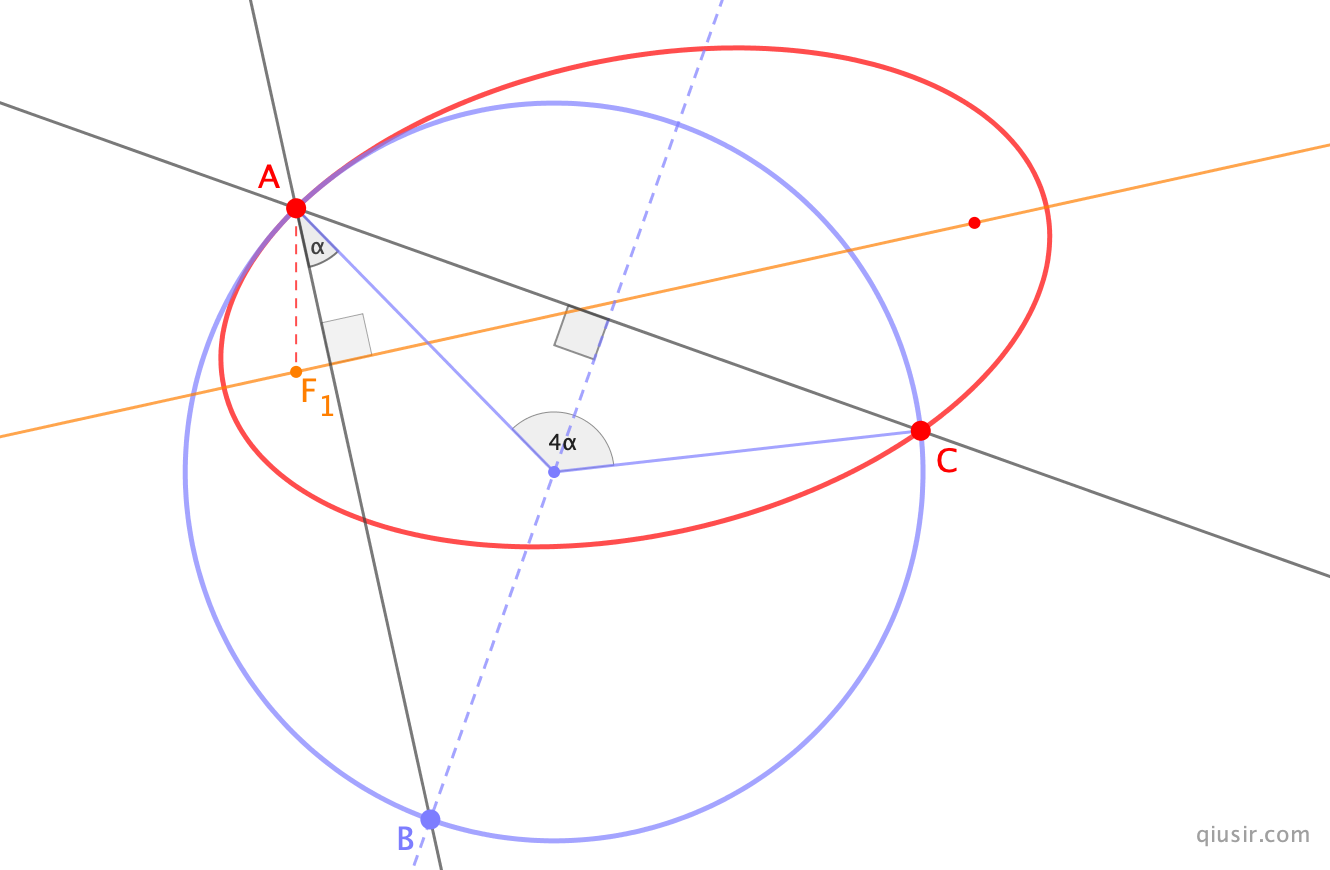### 3 Responses to “圆锥曲线与密切圆交点几何性质”

1. qiusir Says:正是从日本的这位同行分享的图片的到启发，更应该感谢的还有他那有丰富的资料，有很多我要好好学习的地方：https://date-physics.jimdofree.com2. qiusir Says:

Y.S.@YS55749378
去年の京大物理でθ=60°の場合が出題されてましたね
証明はこんな感じですかね3. qiusir Says:

非等速円運動の落下点の角度は、発射点の角度の3倍になる
https://ushitora.net/archives/3156